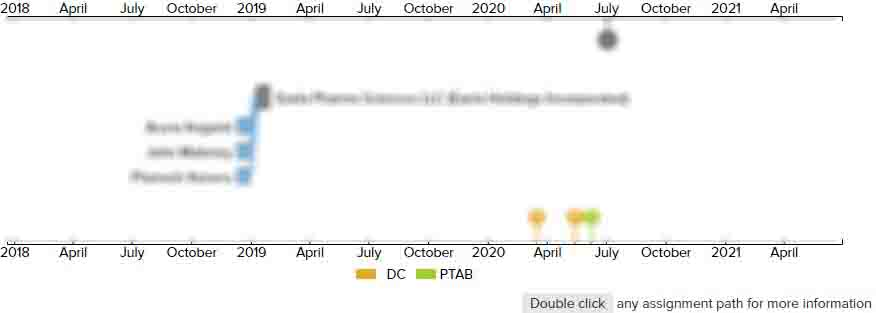# Battery remaining capacity measuring apparatus

• US 5,872,453 A
• Filed: 09/04/1996
• Issued: 02/16/1999
• Est. Priority Date: 07/25/1995
• Status: Expired due to Fees
##### First Claim
Patent Images

1. An apparatus for determining a remaining capacity on a battery to be connected to a load in a circuit, said apparatus comprising:

• a voltage sensor for detecting a voltage value of said battery;

a current sensor for detecting a current value flowing in said load in a closed condition of said circuit where said battery is electrically connected to said load;

a temperature sensor for sensing a temperature of said battery;

a voltage estimate unit for estimating a present battery voltage (Vn) corresponding to a standard current value in accordance with a voltage-current approximate linear function which can be obtained by a plurality of voltage values and current values detected by said voltage sensor and said current sensor in the closed condition of said circuit;

a remaining capacity calculation unit for calculating the remaining capacity corresponding to the present battery voltage (Vn) in accordance with a line pattern (C) between a maximum voltage (VF) and a minimum voltage (VE), said maximum voltage corresponding to a battery voltage detected by said voltage sensor in an opened condition of said circuit where said battery is disconnected with said load, said minimum voltage corresponding to another battery voltage in case of 0% in remaining capacity in accordance with said maximum voltage;

a temperature discriminating unit discriminating whether the sensed temperature is within a predetermined temperature range or is less than or greater than the predetermined temperature range;

a high temperature pattern calculation unit of replacing the line pattern of said remaining capacity calculation unit with a high temperature line pattern (A) when the sensed temperature is greater than the predetermined temperature range; and

a low temperature pattern calculation unit for replacing the line pattern with a low temperature line pattern (B) when the sensed temperature is less than the predetermined temperature range;

wherein said high temperature line pattern (A) is between a maximum voltage for high temperature (VFH), which is obtained by adding a first correction value to the maximum voltage (VF) at said high temperature calculation pattern calculation unit, and a minimum voltage for high temperature (VEH), which is obtained by subtracting a second correction value from the minimum voltage (VE) of the line pattern (C) at said high temperature pattern calculation unit; and

said low temperature line pattern (B) is between a maximum voltage for low temperature (VFL), which is obtained by subtracting a third correction value from the maximum voltage (VF) at said low temperature pattern calculation unit, and a minimum voltage for low temperature (VEL), which is obtained by adding a fourth correction value to the minimum voltage (VE) of the line pattern (C) at said low temperature pattern calculation unit.

• ##### 1 Assignment
Timeline View
Assignment View×
×
×